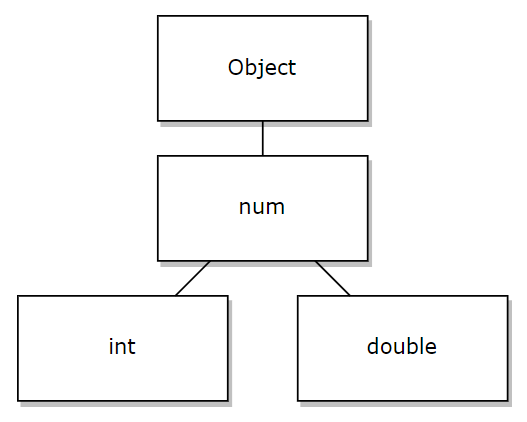## 1. int 和 double

``````void main(List<String> args) {
int age = 10;
print(age); // 输出 10
}``````

``````void main(List<String> args) {
double price = 5.5;
print(price); // 输出 5.5
}``````

``````void main(List<String> args) {
// 1. 把 int 字面量 10 赋值给 double 类型的变量 price
double price = 10;
// 2. 输出 price 的值，发现变成了包含小数位的 double 类型的值：10.0
print(price);
}``````

## 2. 父类型 num

``````void main(List<String> args) {
// 定义 num 类型的变量 a 和 b，其中：
// 为变量 a 赋值整数 10
num a = 10;
// 为变量 b 赋值带小数的数字 1.1
num b = 1.1;
}``````

``````void main(List<String> args) {
// 1. 定义数字类型的变量 price，具体是小数还是整数不明确
num price;

// 2. 为 price 赋值为整数 10
price = 10;
print(price); // 输出 10

// 3. 为 price 赋值为带小数的数字 1.1
price = 1.1;
print(price); // 输出 1.1
}``````

## 3. int 与 double 进行运算

`int` 类型的字面量与 `double` 类型的字面量进行运算，最终得到的结果为 `double` 类型，示例代码如下：

``````void main(List<String> args) {
num result = 10 + 1.1;
print(result); // 输出 11.1

// 注意：Dart 中的 is 是类型判断操作符，用来判断左侧的数据是否为右侧的类型，语法如下：
// 数据 is 类型
// 如果返回 true，则表示左侧数据是右侧类型；如果返回 false，则表示左侧数据不是右侧类型。
print(result is int); // 输出 false，表示运算的结果不是 int 类型
print(result is double); // 输出 true，表示运算的结果是 double 类型
}``````

## 4. 字符串转数字

``````void main(List<String> args) {
int a = int.parse('10');
print(a); // 输出 10

double b = double.parse('1.1');
print(b); // 输出 1.1

num c = num.parse('20');
print(c); // 输出 20
print(c is int); // 输出 true

num d = num.parse('2.2');
print(d); // 输出 2.2
print(d is double); // 输出 true
}``````

``````void main(List<String> args) {
// 1. 如果转换成功，则转换的结果是预期的数字
int? a = int.tryParse('10');
print(a); // 输出 10

// 2. 如果转换失败，则转换的结果是 null，从而防止程序运行异常的问题
// 注意：这里的 int? 表示"可空 int 类型"：表示变量的值既可以是 int 整数，也可以是 null
int? b = int.tryParse('10全大补汤');
print(b); // 输出 null
}``````

``````void main(List<String> args) {
// Unhandled exception:
// FormatException: Invalid radix-10 number (at character 1)
// 1.1
// ^
int c = int.parse('1.1');
print(c);
}``````

``````void main(List<String> args) {
num a = num.parse('10');
print(a); // 输出 10
print(a is int); // 输出 true

num b = num.parse('1.1');
print(b); // 输出 1.1
print(b is double); // 输出 true
}``````

## 5. 数字转字符串

``````void main(List<String> args) {
int a = 10;
print(a.toString()); // 输出字符串 10
// toStringAsFixed(n) 函数用来指定小数点后保留多少位小数，n 是数字
print(a.toStringAsFixed(2)); // 转为字符串并保留两位小数，输出字符串 10.00

double b = 1.1;
print(b.toString()); // 输出 1.1
print(b.toStringAsFixed(2)); // 转为字符串并保留两位小数，输出字符串 1.10

num c = 4.567;
num d = 1.234;
// toStringAsFixed() 在保留小数时，会进行四舍五入
print(c.toStringAsFixed(1)); // 转为字符串并保留一位小数，输出字符串 4.6
print(d.toStringAsFixed(1)); // 转为字符串并保留一位小数，输出字符串 1.2
}``````

## 6. 其它常用函数

1. `abs()` 求绝对值
2. `ceil()` 上取整
3. `floor()` 下取整
4. `round()` 四舍五入
5. `toInt()` 把数字转为 int 类型
6. `toDouble()` 把数字转为 double 类型
``````void main(List<String> args) {
// abs() 求绝对值
int a = -10;
print(a.abs()); // 输出 10

// ceil() 上取整
double b = 10.2;
print(b.ceil()); // 输出 11

// floor() 下取整
double c = 10.99;
print(c.floor()); // 输出 10

// round() 四舍五入
double d = 8.4;
double e = 8.5;
print(d.round()); // 输出 8
print(e.round()); // 输出 9

// toInt() 把数字转为 int 类型
// 注意：直接去掉小数位，不会进行四舍五入
double f = 3.14;
double g = 7.98;
print(f.toInt()); // 输出 3
print(g.toInt()); // 输出 7

// toDouble() 把数字转为 double 类型
int h = 3;
print(h.toDouble()); // 输出 3.0
}``````

## 2条评论

• ### 天成

彬哥开始研究dart是准备去干flutter了吗 嘿嘿

• ### 泥巴巴

不会的，前端还没研究明白呢，呜呜呜